# Distribution of zeros of L-functions

For ease of notation we will phrase everything in terms of the Riemann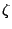-function, with the understanding that all statements hold for general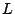-functions with the obvious modifications.

Assume the Riemann Hypothesis, let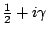denote a nontrivial zero of the-function with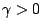, and write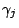for the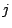th zero, ordered with increasing imaginary part and repeated according to their multiplicity. Let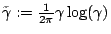, so that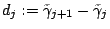has average value of 1. An important problem is establishing various statistical properties of the sequence of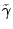.

The pioneering work of Montgomery [49 #2590] on the pair correlation of zeros of the-function, work of Hejhal [ MR 96d:11093], and Rudnick and Sarnak [ MR 97f:11074], on higher correlations of zeros, and extensive numerical calculations of Odlyzko (see [ MR 88d:11082] and [unpublished work] available on his web page), give persuasive evidence of the following:

The GUE Conjecture The (suitably rescaled) zeros of the Riemann-function are distributed like the eigenvalues of large random matrices from the Gaussian Unitary Ensemble.

The conjecture has yet to be stated in a precise form. See The GUE hypothesis for a discussion. However, any reasonable form of the conjecture makes a prediction of the correlation functions of the zeros and the distribution of the neighbor spacings of the zeros, as well as various other statistics. Thus, the GUE conjecture is a powerful tool for making and testing conjectures about the-function, and for shedding new light on a variety of long-standing questions. See the discussions of mean values and value distribution for more examples of how this conjecture relates to classical objects studied in number theory.

Back to the main index for L-functions and Random Matrix Theory.